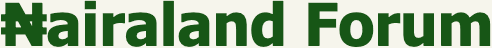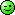Welcome, Guest: Join Nairaland / LOGIN! / Trending / Recent / New
Stats: 2,796,162 members, 6,679,854 topics. Date: Tuesday, 18 January 2022 at 12:29 AM

How To Solve Maths Problems - Education - Nairaland

 How To Solve Maths Problems by samzyworld(m): 11:22am On Jul 04, 2014 Although math problems may be solved indifferent ways, a general method to visualizing,approaching and solving math problems isoutlined in the steps found here. www.samzyworld.com Determine what kind of math you arehaving difficulty with . Is it multiplyingfractions? Solving quadratic equations? Knowingwhere you need more knowledge is key forfocusing your studying.Review. Most math textbooks have a lesson toread, covering new concepts directly beforeeach problem set. If you are having trouble withthe newest formula or method, that is where tostart.Seek help if necessary. Asking a teacher,parent or mathematically gifted friend forhelp is often the best way to receive directguidance and have your questions quicklyanswered.There are many websites and youtube videosthat offer free tutorials or lessons in basicmath concepts. Consider visiting one topractice or quickly reference a formula.Begin to solve the problem. Now that you'vereviewed, it's time to apply your skills.Determine what the problem is asking you tosolve. There is a big difference betweenbeing asked to find the cosine and the sine.Read the directions carefully.Guess and check, "Hmm, I guess it is ___, sothen it would be ___. I'll see whether thatworks."Use objects, manipulatives, to model theproblemUse logical reasoning: "If this is ____, thenthat would give me ____ ..." -- or the negativeversion, "If not this ____, then it's not that ____" ...Look for a pattern -- how series or a sequencechanges from one member (element) of alist, back to the previous, and also forward tothe next member.Do a process -- act it out -- i.e.: experiment asin physical or real world problems.Work backward -- reverse the processes of apossible solution to see whether it mayconnectClassify the kind of problem, process, orpattern it fits.What do I not know or have (Ask thisquestion: "Can I find an intermediate steptoward solving this, for the situation?"Re: How To Solve Maths Problems by samzyworld(m): 11:24am On Jul 04, 2014 Make multiple representations( mathematical models/math-models),approaching your problem; here areexamples of several forms ofrepresentation :Verbal. Write your own description of theproblem (in your own words).Gather data -- using tally marks to keep thecountx,y chart/table. Often a table can be made ofdata in columns (x,y) and rows (for example:money made on sales of candy each week).Drawing or diagramming. For example: drawthe described physical problem situation(possibly involving two dimensional sketch, ageometric figure, or perhaps trigonometry).Mapping (if that applies).Graphing. Called models, many types ofrelations and process dynamics may begraphed in mathematical, physical,biological, social and information systemsdata. Graphs may be of numerous types, buta basic is to graph paired information( pairwise relations), such as growth or decayover a period of time:Bar graph;Pictograph;Cartesian points (x,y) graphed in theCartesian plane.Line graph joining the results of data (suchas growth) over time;Time-line -- a special graph used for[historical] information over time;A circle graph/pie chart = total 1 whole or100% (a kind of "pizza math"Scatter plot, point graph of data (oneexample is a distribution of data pairs);Trend line/simple linear regression (thecentral tendency compare to the median oraverage) -- a linear representation of paireddata, shows the "average" line, in a dataarray/distribution;Note: A multivariate linear regression hastwo are more variables (for example, threevariables: (1st variable) measure thegrowth of plant seedlings, (2nd variable)for each of two experimentaltemperatures, (3rd variable) over the sametime period(s));Conjecture/structure a function -- anotherkind of model perhaps, y = f(x) = ______(using a mathematics, physics or geometricequation or formula) may be constructed tofit the problem parameters (facets);Check your data over the domain of x andthe range of y (decide parameters); ask: "Isit linear or non-linear?"Graph your proposed function.6Double-check your work. Did you drop anydecimal places or the decimal point?Accidentally write the numerator as yourdenominator? Now is the time to find out!7Check your answer for reasonableness,accuracy and repeatability .If your answer doesn't match the correctone, go back and check your work for whereyou may have made a mistake.